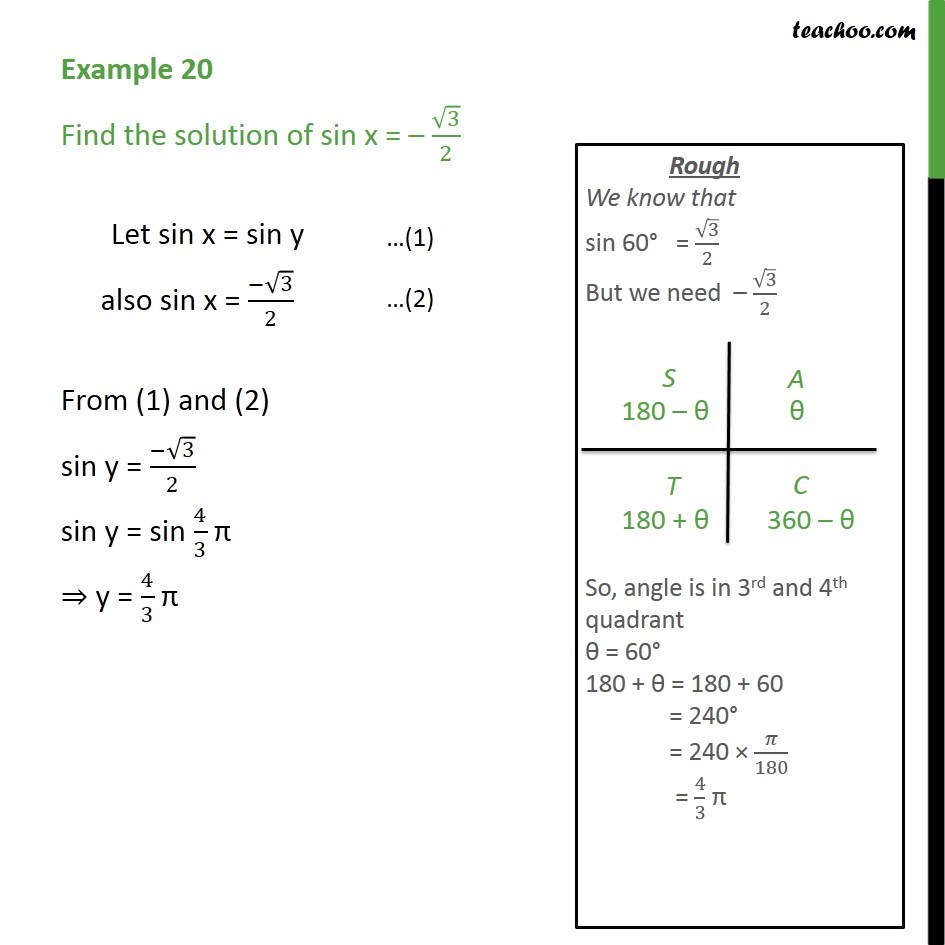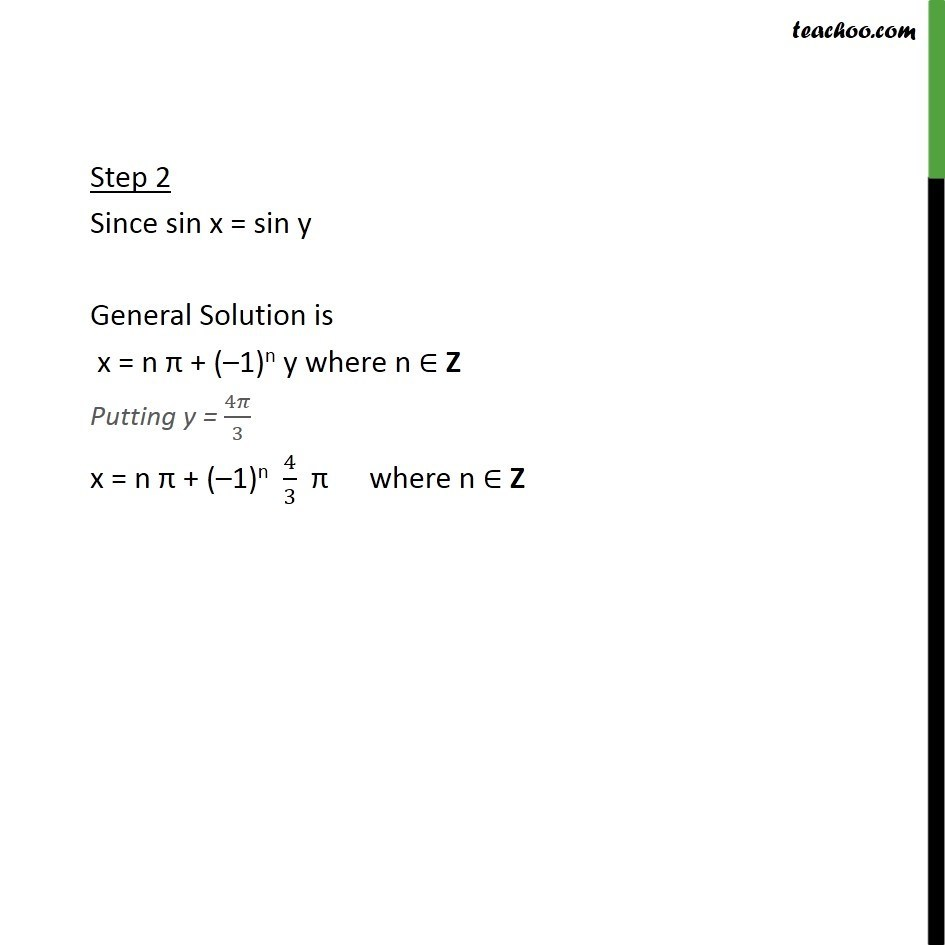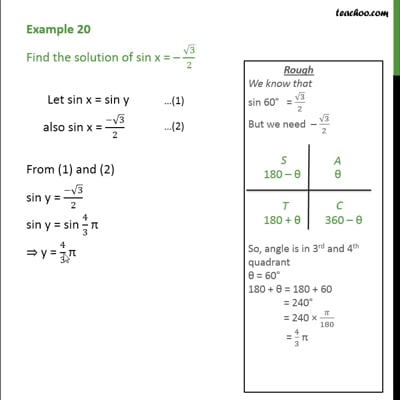Examples

Chapter 3 Class 11 Trigonometric Functions
Serial order wiseThis video is only available for Teachoo black users

Solve all your doubts with Teachoo Black (new monthly pack available now!)

### Transcript

Example 20 Find the solution of sin x = – √3/2 Let sin x = sin y also sin x = (−√3)/2 From (1) and (2) sin y = (−√3)/2 sin y = sin 4/3 π ⇒ y = 4/3 π Step 2 Since sin x = sin y General Solution is x = n π + (–1)n y where n ∈ Z Putting y = 4𝜋/3 x = n π + (–1)n 4/3 π where n ∈ Z